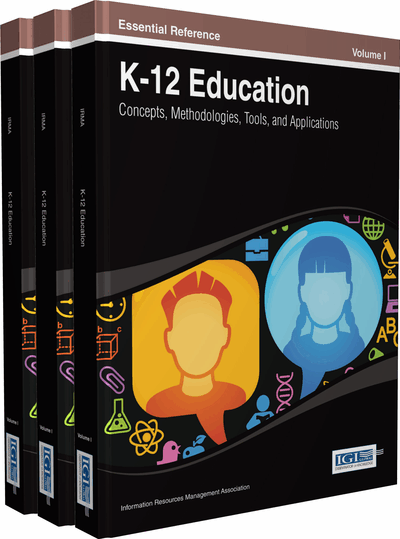# Solving Equations is All about Balance: Using Virtual Manipulatives in the Middle School Classroom

Robin Magruder, Margaret Mohr-Schroeder
DOI: 10.4018/978-1-4666-4502-8.ch059
OnDemand:
(Individual Chapters)
Available
\$37.50
No Current Special Offers

## Abstract

Virtual manipulatives provide benefits to students as they encounter the Common Core State Standards for Mathematics content and practice standards. The National Library of Virtual Manipulatives provides an abundant supply of virtual manipulatives for all K-12 grades and mathematics content areas. Specifically, in this chapter, the authors explore the use of Algebra Balance Scale manipulatives in a middle school mathematics classroom. Using virtual manipulatives increases understanding of the equal sign, algebraic symbols, and increases procedural and conceptual knowledge. In this chapter, the authors demonstrate how using virtual manipulatives helped middle school students to meet grade level content standards for solving equations in one variable. Additionally, practice standards, such as making sense of problems and persevering to solve them, modeling with mathematics, and using tools strategically through the use of virtual manipulatives are addressed. They conclude by considering practical issues related to incorporating virtual manipulatives in mathematics classrooms.
Chapter Preview
Top

## Background

The Common Core State Standards for Mathematics (CCSSM) include grade-specific content standards and over-arching mathematical practice standards. An important theme of the algebra strand of the CCSSM is that middle school students are expected to reason about and solve one-variable equations (CCSSO, 2011). For example, a sixth grade-specific standard (6.EE.7) states students should solve real-world and mathematical problems by writing and solving equations of the form x + p = q and px= q for cases in which p, q, and x are all nonnegative rational numbers. A similar example at the eighth grade level (8.EE.7) states students should solve linear equations in one variable. Additionally, according to the sixth-grade standard 6.NS.5, students should understand that positive and negative numbers are used together to describe quantities having opposite directions and values.

Students often face challenges in mathematics content, especially when trying to make sense of abstract concepts such as solving one-variable equations. Specifically, researchers have identified three common challenges that students often face when attempting to solve equations:

• 1.

Lack of understanding of the equal sign (Knuth, Stephens, McNeil, & Alibali, 2006).

• 2.

Lack of symbolic understanding within an equation (Kilpatrick & Izsak, 2008; Poon & Leung, 2010).

• 3.

Reliance on procedural knowledge without conceptual understanding (Capraro & Joffrion, 2006; Siegler, 2003; Star, 2005).

Students can increase their understanding of solving equations in one-variable by interacting with representations found in virtual manipulatives (Durmus & Karakirik, 2006; Sarama & Clements, 2009).

### Meaning of the Equal Sign

Research dating as far back as the 1970s indicates that many students have an incomplete understanding of the meaning of the equal sign (Kieran, 1992; Knuth et al., 2006; Rojano, 2002). Although the equal sign is a ubiquitous element in mathematics classrooms, little time is devoted to its meaning (Knuth et al., 2006). In their seminal work on student understanding of mathematics, Kilpatrick, Swafford, and Findell (2001) described student misconceptions related to the equal sign. Many students described the equal sign as a separation of the question and answer. Other students described a directional symbol, stating that they should work from left to right to solve problems.

Knuth et al. (2006) revealed student misconceptions of the equal sign as they conducted a quantitative study of middle school students (n=177). A majority of students described the equal sign as operational, expecting or announcing an answer. Students had difficulty solving equations with variables on both sides because they were unsure how to proceed based on their limited understanding of the equal sign. A limitation of this study was a lack of exploration of teaching methods, which led students to their understandings of the equal sign.

## Complete Chapter List

Search this Book:
Reset